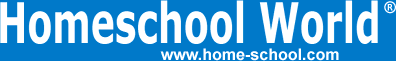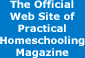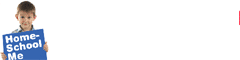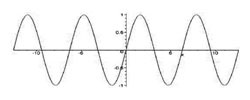Home Curriculum Catalog Articles Contests Events Groups Forum News ContactGetting Started Preschool/K Elementary Middle School High School College & Career Prep Special Needs Group Activities Research FAQs# Advanced Math: Trig, PreCalc, and more!

By Bill Pride
Printed in Practical Homeschooling #68, 2005.Advanced Math: the bridge to Calculus.I'd like every homeschooled student and parent reading this article to plan on including Advanced Math in their homeschool program.

Why? After all you don't need advanced math to graduate high school. You don't need it yet for the SAT. If you're absolutely certain you are going to be a journalist, or a historian, or a truck driver, you're probably never going to use advanced math in your entire professional career.

But I can still think of at least four reasons to consider including advanced math in your high-school plans:

1. Would you like to increase your math score on the ACT and thus make yourself a better candidate for college? The ACT has 12 questions on coordinate geometry and 12 questions on trigonometry. That's 24 questions out of 71 you will stare at uncomprehendingly if you didn't take Advanced Math.

3. If you are thinking of any scientific, technical, medical, engineering, or math oriented career, you will need this course, probably just to get accepted into their college program.

4. Because it's cool!

Advanced Math is called by many names, the three most common of which are Advanced Mathematics, Pre-Calculus, and Trigonometry. Trigonometry is the study of the trigonometric functions-sine, cosine, tangent, cosecant, secant, and cotangent-their graphs, and the relationships between the functions (trigonometric identities). In trig courses you spend a lot of time analyzing triangles, especially right triangles. Finally you learn about the inverse trig functions and polar coordinates.

Trigonometry is just one part of precalculus. Precalculus studies the trig functions plus analytical geometry: linear functions and systems of linear equations, polynomials, rational functions, exponential functions, logarithmic functions, and conic sections (shapes you get by cutting a cone at various angles-circles, ellipses, parabolas, and hyperbolas).

Advanced Math is all that and more. Exactly how much more depends on the school system or the textbook publisher making up the scope and sequence (the list of topics the course covers).

So trig is the smallest course, precalculus is medium-size, and advanced math is the whole deal, up to and probably including a bit of calculus.

Beware of Dumpster Math

Because Advanced Math is the last course you will take before moving on to college level mathematics, some publishers use it as the dumpster for everything they think you should learn but haven't been able to shoehorn into their previous textbooks. So you get bits of linear algebra or probability, or on the other extreme, geometry. Does this help? Not really. You can't get more than the bare bones of any of these subjects in just one unit of a one year advanced math course. It's better to learn your trigonometry and coordinate geometry thoroughly than to get a smorgasbord of topics that you learn superficially.

Make It Easier... Take Two Years

If your student has handled Algebra 1 and 2 and Geometry, he or she can manage Advanced Math. If Algebra and geometry were difficult for your student, instead of giving up, try teaching Advanced Math at an easier pace.

Some people, split advanced math into two parts and teach the trigonometry in one year and the analytical geometry in another. You can do this if you start with algebra in seventh grade. Your study sequence would then be:

Grade 8 - Algebra 2 or Geometry

Grade 9 - Geometry or Algebra 2

What Ought I to Be Learning?

Advanced Math has one primary reason for existing: bridging the gap between Algebra/Geometry and Calculus. As a distant second it should prepare you for the ACT math sections. Anything else it includes is just a bonus.

You need to know all of trigonometry. You'll be visiting your sines and cosines again in a big way in Calculus, so you need to know them cold. You need to know all the basic functions and relations I listed earlier.

Why these particular functions? In physics many of these functions are used to describe some sort of real world motion:

• Linear (y = mx + b) - you cut your teeth in Physics studying motion in a straight line

• Parabola (y = ax2 + bx + c) - plotting the trajectory of a cannon ball

• Circle (x2 + y2 = r2) - analyzing the forces on a car going around a curve

• Ellipse (x2 / a2 + y2 / b2 = 1) - computing the orbit of a planet

• Hyperbola (x2 / a2 - y2 / b2 = 1) - figuring the path of an object that has a near miss with a planet

• Sine or cosine - predicting the motion of a piston attached to a flywheel on a steam engine

Trig functions are also used to describe sound waves and other wavelike signals. This is because the graph of y = sin(x) looks like a wave.Other functions describe the behavior of chemical or biological phenomena:

• Exponential function on x - population growth

• Exponential function on -x - radioactive decay

If you want to know where something is going, where it came from, how it accelerated or decelerated, how much time and energy this all took... or if you want to understand how waves behave... you need these basic functions.

How do I Learn or Teach Advanced Math?

Lots of help is available for teaching advanced math. Here are a few of the most common homeschool resources:

Workbooks and textbooks. When you get to advanced math, your options for homeschool textbooks begin to narrow. A Beka (www.abeka.com) has separate Trigonometry and Analytical Geometry textbook sets. BJU Press (www.bjup.com) has Precalculus. Saxon Math (www.hmhco.com/homeschool/math) has very comprehensive Advanced Math and Calculus.

DVD instruction. These programs go beyond textbooks by offering an instructor teaching and working problems on video. Accompanying textbooks or worksheets and answer keys are also included. Chalk Dust (www.chalkdust.com) and Math-U-See (www.mathusee.com) Pre-Calculus fall into this category.

Software is interactive. It provides demonstrations and grades problems. The disadvantage of software is that you have to sit in front of the computer: not good for more than one kid at a time. Advanced Math software is available from a number of publishers, including Grasp Math (www.sieducation.com). DIVE Advanced Math software from Genesis Science (www.diveintomath.com) works through all the problems in the Saxon textbook. MathMedia Educational Software (www.mathmedia.com) sells a set of seven Advanced Math modules as well.

Online courses are also interactive, but add tutors as well so kids can email a teacher with their questions. Online courses tend to be more expensive and advanced math online courses are pretty hard to find. The best place to look are universities that offer online courses: for example, University of Washington (www.pce.uw.edu/online.aspx) or University of Nebraska-Lincoln Independent Study High School (highschool.nebraska.edu).

Study guides. The people who make the Quick Study Guides we sell in our catalog also have guides for Trigonometry and Pre-Calculus.Terms of Use   Privacy Policy Copyright ©1993-2023 Home Life, Inc.